# Geometrical Optics

NOTE

This manual describes the laboratory experiment used during the 1996 - 1997 academic year. Significant changes have been made since then, and the manual used during the current academic year is in NOT available yet on the WEB. Hardcopies can be purchased at the bookstore.

## Purpose

To verify the thin lens equation for both a biconvex lens and a concave mirror, and to construct simple optical devices using various combinations of lenses.

## Introduction

Various types of images are produced by lenses and mirrors, depending on the position of the object relative to the lens (mirror) and its focal point. In the following discussions, we use the attributes real or virtual, erect or inverted, and reduced or magnified to describe these images. The fundamental distinction between real and virtual images is that light rays converge to form a real image, while light rays diverge from a virtual image; real images may be projected onto a screen while virtual images must be viewed through a lens system such as the eye.

In this experiment, we use a simple "optical bench," a track upon which various combinations of objects and lenses (or mirrors) can be mounted. The separation of lens (or mirror) and object, denoted by u , and lens (or mirror) and image, denoted by v , can be measured by noting the relative position of each on the optical bench. If the focal length of a lens (mirror) is f, we may use the optical bench to check if the thin lens equation

1/u + 1/v = 1/f (12.1)

is satisfied for a given u and v. The bench will also be used to construct a compound microscope and two types of telescopes: astronomical and terrestrial.

## Prelab Homework

The prelab homework must be done at home and handed to the lab TA before you start the lab. Read the instructions for this lab.

Questions

• Determine the locations of the images of the objects(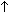) drawn below.

• Describe the images using the terms magnified or reduced, erect or inverted and real or virtual.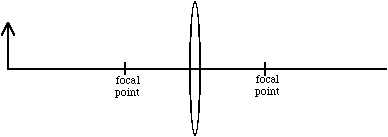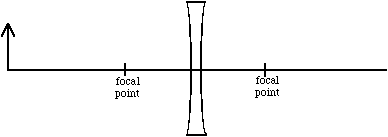## Experiment

### Part I: The Focal Length of a Converging Lens

For this part, use the large bi-convex lens, an "object" (lamp), and an imaging screen. See Figure 12.1 for set up.Figure 12.1

Assuming the thin lens equation (12.1) is satisfied, we may use the optical bench to measure u and v for several images in order to obtain a value for the focal length of the biconvex lens. The sign convention for equation (12.1) is that v is taken to be positive to the right of the lens and negative to the left.

Procedure

• Place the object on the optical bench near one end, and place the screen at the opposite end, for example at 0 cm and 100 cm.

• Slide the lens along the bench between object and screen and find all (two or less) positions where the lens produces a sharp image on the screen.

• For each position of the lens, record u and v in a table and describe the image using the terms real or virtual, erect or inverted, and magnified or reduced.

• Move the screen about 10 cm closer to the object and try to find all images again. Record u and v. and the image attributes.

• Repeat, moving the screen closer to the object by 10 cm until there are no more sharp images on the screen. At this point the two images have come together until there is only one. There are no more images for closer separations.

### Part II: The Focal Length of a Concave Mirror

For this part use the concave mirror, the lamp, and the imaging screen. The thin lens equation is still obeyed, only u and v are defined as in figure 12.2, where v is taken to be negative behind the mirror.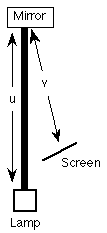Figure 12.2

Procedure

• Place the mirror at one end of the optical bench as in figure 12.3. It will remain there the whole time. At the opposite end put the lamp.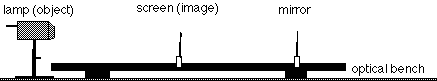Figure 12.3

• Now slide the screen along the rule and find all the positions where the mirror produces a sharp image on the screen. There may be some difficulty in locating the image because the screen will block the rays from the object. You will have to aim the object slightly to one side (or angle it up) and hold the screen next to the optical bench.

• In the table on your lab sheet record the values of u and v and the attributes of the image.

• Next move the object 10 cm closer to the mirror, finding again the place(s) where image(s) are formed. Record u and v and the image attributes.

• Repeat these observations moving the object each time 10 cm closer to the mirror, until it is no longer possible to form an image on the screen.

### Part III: The Compound Microscope

The compound microscope uses two lenses. The one closest to the object is called the objective lens; the one closest to the observer is called the eyepiece.

The objective lens has a small focal length, possibly only a few millimeters. The object is placed slightly beyond the focal point of the objective in such a location as to form an enlarged real image inside the focal point of the eyepiece.

The eyepiece is then usually adjusted to form an enlarged virtual image about 25 cm from the viewer, as 25 cm is the standard value for the "near point" of the eye, or the closest point upon which the eye can focus. By having the image at the near point, its apparent size is maximized. The total magnification M of the microscope is the product of the lateral magnifications of the objective lens m1 and of the eyepiece m2 (i.e., |M| < 1 ==> image reduction, if M is positive the image is erect, and if M is negative the image is inverted.),

M = m1 m2. (12.2)

If the eyepiece forms a virtual image 25 cm behind the eyepiece, the image distance v is equal to -25 cm. We then may use the thin lens equation to write the magnification as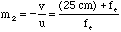, (12.3)

where fe = 20cm is the focal length of the eyepiece.

We will use the above equation for m2 along with a measured value for m1, to investigate the total magnification M of the microscope.

Procedure

• Construct a compound microscope on the optical bench as in figure 12.3 by combining the short focal length bi-convex lens with the large bi-convex lens used in the first section.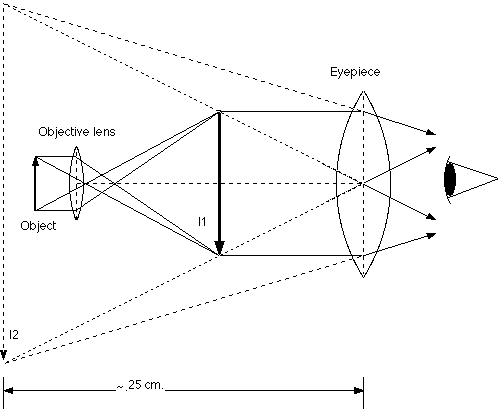Figure 12.3

• Tape a resistor to the side of the imaging screen and illuminate it with a desk lamp. Adjust the positions of the two lenses so that you obtain the best magnified focused image.

• Holding the ruler between your eye and the eyepiece, make a rough measurement of the apparent size of the object.

• Keeping the distance between the ruler and the object(and your eye) the same, remove the object from the microscope and estimate its actual size (as in Figure 12.4). The ratio of these sizes gives an estimate of the magnification M for the lens system.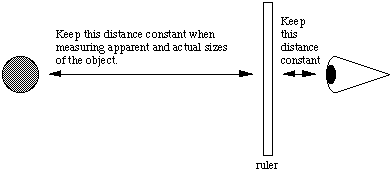Figure 12.4

### Part IV: The Telescope

A. The Astronomical Refracting Telescope

For an astronomical telescope, the objective lens is always a lens with a long focal length. The objective lens produces a real image at its focal point. This image is magnified with the eyepiece. In this case, the eyepiece is usually adjusted so that the virtual image is at infinity, in this case a piece of paper across the room, but it may be adjusted to be closer.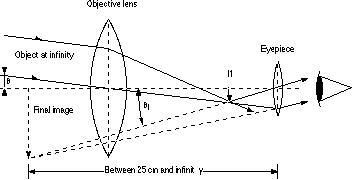Figure12.5

Because theta and theta1 are small, they can be approximated as tan(theta) and tan(theta1), respectively. If h is the height of the real image I1 formed by the objective lens, then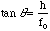, (12.3)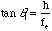, (12.4)

and so,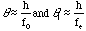,

where fo and fe are the focal lengths of the objective and eyepiece lenses, respectively. In addition, fo is the distance from the objective lens to I1 (because the image is at infinity) and fe is the distance from the eyepiece to I1 if the eyepiece is adjusted to give an image at infinity. The angular magnification then is,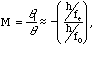(12.5)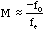. (12.6)

Procedure for Astronomical Telescope

• Construct an astronomical telescope on the optical bench as in figure 12.5 using the small bi-convex lens for the eyepiece and the large bi-convex lens for the objective.

• Attach the lenses to the optical bench such that the distance between the lenses is about equal to the sum of their focal lengths.

• Focus on a distant target using the telescope and estimate its apparent size. A good object to help in your estimation is a ruler or something with a regular pattern of lines such as the window blinds.

• Estimate the size of the object when you look directly at it with the naked eye. Also record what type of image you see (upright or inverted, left-to-right inversion or not).

B. The Terrestrial Telescope (i.e. Opera Glass)

As in the astronomical telescope, the terrestrial telescope utilizes a long focal length lens and a short focal length eyepiece to produce a magnified image of a distant object. The magnification is again given by M = fo/fe. However, the eyepiece is a "meniscus" or concave lens rather than a convex lens, and things are somewhat different for this kind of telescope.

Procedure

• Construct a terrestrial telescope on the optical bench by replacing the eyepiece from the astronomical telescope with a meniscus lens like that shown in figure 12.7. Use the same objective lens as in the astronomical telescope.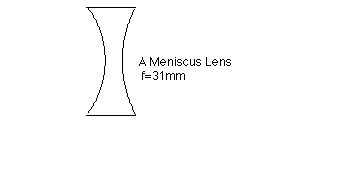Figure 12.7

• Put the eyepiece at the very end of the optical bench.

• Aim at a distant target and focus the telescope by sliding the objective back and forth until the image is sharply focused.

• In the same way as before, estimate the magnification of the telescope by comparing the image seen in the telescope with the image seen with the naked eye. Is the image erect or inverted, direct or left-to-right inverted?

## Data Analysis

### Part I- The Bi-convex Lens

• Using the data from your table, graph u vs. v, on linear paper and draw a smooth curve through the points. From the equation, if u becomes very large, what happens to v? Also if v becomes very large, what happens to u? Compare these results with your graph.

• Draw a graph of 1/u vs. 1/v. The graph should be a line of slope -1 and the y-intercept is 1/f. The graph is of the thin lens equation,

1/u + 1/v = 1/f (12.1)

• Using the graph in 2) above, estimate the focal length of the lens. Using the data in your table, calculate the focal length f and its uncertainty for each pair of values, u and v. Compute the average focal length for the lens and compare this to the graphical estimate from 2) above. This value will be useful later on in the experiment.

• For the bi-convex lens, what is the closest that the image and object can come to each other, and how did you deduce this from the graph?
• Objects which are closer to the lens than the focal point do not produce real images, so where do the object and image appear on the graph of u vs. v (indicate on your graph from 1))?
• Describe the images seen using the terms magnified or reduced, erect or inverted, and real or virtual for the two cases below:
• When the lens is closer to the object that to the image (v>u).
• When the lens is closer to the image than to the object (u>v).

### Part II- The Concave Mirror

• Make a graph of u vs. v and1/u vs. 1/v for the concave mirror. Label the axes and draw smooth curves through the points. Estimate the focal length of the mirror from the graph.

• Using the data in your table, calculate the focal length f and its uncertainty for each pair of values, u and v.

• Compute the average focal length for the mirror and compare this to the graphical estimate.

• Describe the images seen using the terms magnified or reduced, erect or inverted, and real or virtual for the two cases below:
• when the mirror is closer to the object than to the image (v > u).
• when the mirror is closer to the image than to the object (u > v).

### Part III- The Compound Microscope

• Is the image erect or inverted? Is there a left-to-right inversion (i.e.-left and right switched, as in a mirror?)?
• The quality of the image is probably quite poor. Describe what is wrong with it.

• Given the parameters fe,fo, and u (object distance from first lens), find an expression for M, the magnification of the microscope. Leave the expression in terms of the above quantities.

### Parts IVA&B-The Astronomical and Terrestrial Telescopes

• Compare your estimated value of M for the astronomical telescope to the theoretical value M = fo/fe and answer the following questions:
• Is the image erect or inverted?
• Is there a left-for-right inversion?

• Answer the above questions for the terrestrial telescope.

• In terms of the image quality, or "ease of use," are there any important differences between the astronomical and terrestrial telescopes?

The inverted real image

Here is a quick, easy, and perhaps perplexing demonstration you can do at home. There is no doubt that images in the eye are inverted, for this is the result of the crossing of light rays in the optical system of the eye. Why, if the images are upside-down, do we see things the right side up? It is quite possible to read plain print upside-down, and children just beginning to read sometimes choose to do so. A constellation of stars visible in both the northern and southern hemispheres is upside-down in one and not in the other, yet neither observer thinks of himself as anything but the right side up! Just as we "see" the normal, inverted image the right side up, if we produce an image right side up we may expect to "see" it inverted.

Make a pinhole (with a straight pin) in a piece of a file card. Hold the card about seven inches in front of one eye and look at the sky, the ceiling, or a blank wall. Then hold the pin vertically with its head uppermost between the card and your eye. The pinhead is too near to be focused in the ordinary way by the eye, but the rays of light from the hole cause it to cast a shadow on the retina. This shadow is right side up, and so the pinhead is seen upside-down in the hole. If there are several closely spaced holes there will be several shadows, and an inverted pinhead will appear in each hole!